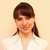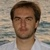# Posts tagged "Excel functions", page 4## How to calculate median in Excel - formula examples

The median is one of the three main measures of central tendency, which is commonly used in statistics for finding the center of a data sample or population. Continue reading## Lookup in Excel: functions and formula examples

Looking up a specific value within a dataset is one of the most common tasks in Excel. And yet, there exists no "universal" lookup formula suited for all situations. In this tutorial, you will find an overview of the most popular Excel Lookup functions with formula examples and in-depth tutorials linked for your reference. Continue reading## Excel LOOKUP function with formula examples

There are a handful of different ways to look up and match in Excel. This tutorial discusses the LOOKUP function that is designed to handle the simplest cases of vertical and horizontal lookup. Continue reading## How to use Excel HYPERLINK function to create different link types

There are many ways to create a hyperlink in Excel. If you plan to insert many identical or similar hyperlinks, the fastest way is to use a Hyperlink formula, which makes it easier to insert, copy and edit hyperlinks. Continue reading## How to Vlookup multiple values in Excel with criteria

When using Microsoft Excel for data analysis, you may often find yourself in situations when you need to get all matches based on a specific id, name, email address or some other unique identifier. An immediate solution that comes to mind is using the Excel VLOOKUP function, but the problem is that it can only return a single match. Continue reading## Excel MID function to extract text from the middle of a string

MID is one of the Text functions that Microsoft Excel provides for manipulating text strings. At the most basic level, it is used to extract a substring from the middle of the text string. The tutorial explains the specificities of the Excel MID function and shows a few creative uses to accomplish challenging tasks. Continue reading## Video: Conditional formatting based on another cell

Learn how to quickly highlight entire rows based on another cell value in Excel. Continue reading## How to use Excel RIGHT function - formula examples

Today our focus is on the RIGHT function, which is designed to return a specified number of characters from the rightmost side of a string. Like other Text functions, RIGHT is very simple and straightforward, nevertheless it has a few unobvious uses that might prove helpful in your work. Continue reading## Excel LEFT function with formula examples

The tutorial shows how to use the LEFT function in Excel to get a substring from the beginning of the text string, extract text before a certain character, force a Left formula to return a number, and more. Continue reading## Excel TEXT function with formula examples

Microsoft Excel is all about numbers, and in your worksheets, you are likely to deal with numbers most of the time. However, there are situations when you do need to convert numbers to text, and it is where the TEXT function steps in. Continue reading## How to use MATCH function in Excel - formula examples

There exist many different Excel lookup functions for finding a certain value in a range of cells, and MATCH is one of them. Basically, it identifies a relative position of an item in a range of cells, however, the Excel MATCH function can do much more than its pure essence. Continue reading## How to use HLOOKUP in Excel with formula examples

The tutorial explains the specificities of the Excel Hlookup function and how to use it most efficiently. Among other things, you will learn Hlookup formulas to search with approximate and exact match, from another sheet and workbook, case-sensitive horizontal lookup, and more. Continue reading## Excel nested IF statement - multiple conditions in a single formula

If someone asks you what Excel function you use most often, what would your answer be? In most cases, it's the Excel IF function. A regular If formula is very straightforward and easy to write. But what if your data requires more elaborate logical tests with multiple conditions? Continue reading## How to compare two cells in Excel (string comparison)

While Excel formulas are always perfectly true, their results may be wrong because some flawed data penetrated into the system. This tutorial will teach you how to automate the tedious and error-prone task of cell comparison and what formulas are best to use in each particular case. Continue reading## How to remove spaces in Excel - leading, trailing, non-breaking

There are several ways to remove spaces in Excel, and this tutorial will help you choose the right technique - delete leading and trailing spaces, eliminate extra spaces between words, get rid of non-breaking white space and non-printing characters. Continue reading## Excel TRIM function - quick way to remove extra spaces

The tutorial demonstrates a few quick and easy ways to trim spaces in Excel – leading, trailing, and excess spaces between words. Continue reading## How to calculate age in Excel: from date of birth, between two dates

There is no special function to calculate age in Excel, however there exist a few different ways to convert date of birth to age. This tutorial will explain the advantages and drawbacks of each way, shows how make a perfect age calculation formula in Excel and tweak it for solving some specific tasks. Continue reading## Using Advanced Filter in Excel - criteria range examples with formulas

In our previous article, we discussed different aspects of Excel Advanced Filter and how to use it to filter rows with AND as well as OR logic. Now that you know the basics, let's have a look at more complex criteria range examples that may prove helpful for your work. Continue reading## Excel SUMPRODUCT function with formula examples

Excel SUMPRODUCT is a remarkably versatile function with many uses. The aim of this tutorial is to reveal the full power of SUMPRODUCT and teach you how to compare arrays in a clever and elegant way, conditionally sum or count cells with multiple criteria, get a weighted average, and more. Continue reading## Excel SUBTOTAL function with formula examples

The tutorial explains the specificities of Excel SUBTOTAL function and shows how to use Subtotal formulas to summarize data in visible cells. Continue reading## How to add, use and remove Subtotals in Excel

Worksheets with a lot of data can often look cluttered and difficult to comprehend. Luckily, Microsoft Excel provides a powerful Subtotal feature that lets you quickly summarize different groups of data and create an outline for your worksheets. Continue reading## How to calculate CAGR (compound annual growth rate) in Excel

The tutorial explains the basics of the Compound Annual Growth Rate and provides a few formulas to calculate CAGR in Excel. Continue reading## Use the new Excel IFS function instead of nested IF

From this short tutorial you'll learn about the new IFS function and see how it simplifies writing nested IF in Excel. You'll also find its syntax and a couple of use cases with examples... Continue reading## How to count words in Excel

Excel doesn't provide any function or built-in tool for counting the number of words in a cell or range. Luckily, we can combine serval functions within a formula to get the desired result. In this tutorial, you will find a few useful formulas to count total or specific words in a cell or range. Continue reading## How to use LEN function in Excel to count characters in a cell

Are you looking for an Excel formula to count characters in a cell? If so, then you have certainly landed up on the right page. This short tutorial will teach you how you can use the LEN function to count characters in Excel, with or without spaces. Continue reading## How to split text string in Excel by comma, space, character or mask

Splitting text from one cell into several cells is the task all Excel users are dealing with once in a while. Today, we are going to take an in-depth look at how you can break strings into different elements using formulas and the Split Text feature. Continue reading## Excel Cumulative Sum - easy way to calculate running total

This short tutorial shows how a usual Excel Sum formula with a clever use of absolute and relative cell references can quickly calculate a running total in your worksheet. Continue reading## Excel SUM formula to total a column, rows or only visible cells

The tutorial shows the most effective ways to use Excel's AutoSum feature and write your own Sum formulas to total a column, rows or selected range. You will also learn how to sum only visible cells, calculate running total and find out why your Sum formula is not working. Continue reading## Excel SWITCH function - the compact form of nested IF statement

This article introduces you to the Excel SWITCH function, describes its syntax and provides a couple of use cases to illustrate how you can simplify writing nested IFs in Excel. Continue reading## How to count unique values in Excel

In this tutorial, you will learn how to count unique values in Excel with formulas, and how to get an automatic count of distinct values in a pivot table. We will also discuss a number of formula examples for counting unique names, texts, numbers, cased-sensitive unique values, and more. Continue reading